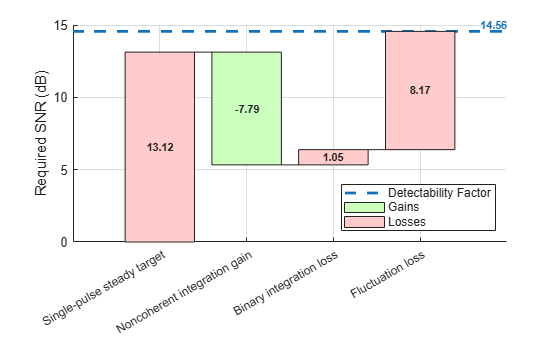Display link budget as waterfall plot

## Syntax

``radarbudgetplot(gl,names)``
``radarbudgetplot(glnames,Parent = hax)``
``hax = radarbudgetplot(___)``

## Description

example

````radarbudgetplot(gl,names)` visualizes a radar link budget as a waterfall chart. A link budget displays the gain or loss `gl` of each link component. The `names` argument specifies the names of the link budget components corresponding to the entries in `gl`.```
````radarbudgetplot(glnames,Parent = hax)` also specifies the plot axes `hax`.```
````hax = radarbudgetplot(___)` returns the plot axes `hax`.```

## Examples

collapse all

Visualize the link budget for a radar system designed to have a probability of detection of 0.9 and a probability of false alarm of${10}^{-6}$. The radar observes a Swerling 1 target and performs M-of-N integration with M = 6 and N = 10.

```Pd = 0.9; Pfa = 1e-6; M = 6; N = 10;```

First, find the integration gain by comparing the detectability of a Swerling 0 target for N pulses versus one pulse.

```det_1 = detectability(Pd,Pfa,1,'Swerling0'); det_N = detectability(Pd,Pfa,N,'Swerling0'); Gain_int = det_N - det_1;```

Next, compute the binary integration loss.

`Loss_bi = binaryintloss(Pd,Pfa,N,M);`

Last, compute the target fluctuation loss.

`Loss_fluct = detectability(Pd,Pfa,N,'Swerling1') - det_N;`

```radarbudgetplot([det_1 Gain_int Loss_bi Loss_fluct], ... {'Single-pulse steady target' 'Noncoherent integration gain' ... 'Binary integration loss' 'Fluctuation loss'})```## Input Arguments

collapse all

Radar gains and losses components, specified as a real-valued vector. Each entry in the vector represents a contribution to the total gains or losses. Units are in dB.

Example: [13.2, -7.8]

Data Types: `double`

Link budget component names, specified as a length-N cell array of character vectors or length-N cell array of strings.

Example: ```{'Single-pulse steady target','Pulse integration gain'}```

Data Types: `char` | `string`

Plot axes, specified as a scalar.

Data Types: `double`

## Output Arguments

collapse all

Plot axes, returned as a scalar.

collapse all

A radar link budget is used to find the received radar signal level based on the transmitted signal level, taking into account all the losses and gains found along the signal path. Together with the noise level measurements, you can use the link budget to calculate the received signal to noise ratio. The `radarbudgetplot` function illustrates the components of the link budget and lets you visualize the radar detectability factor . The radar detectability factor is the minimum SNR required to make a detection with specified probabilities of detection, Pd, and false alarm, Pfa. A waterfall chart shows the contributions of the individual losses and gains present in the radar system to the total power required by the radar to produce a detection.

Once the radar detectability factor is computed, you can use the radar equation to determine the range at which the available SNR for a given target is equal to the radar detectability factor. At ranges where the available SNR exceeds the detectability factor, the radar can make detections with the specified Pd and Pfa. At the ranges where the available SNR is lower than the detectability factor, the radar cannot achieve the required Pd and Pfa.

The actual SNR tells you if the combined gains and losses are sufficient to exceed the required SNR. to declare a detection. For example the required SNR to detect a Swerling 1 target is substantially higher than for a Swerling 0 target.

```Pd = 0.9; Pfa = 1e-6; D0 = detectabilty(Pd,Pfs,1,'Swerling1')```
D0 = 13.1

D1 = 21.1

A Swerling 0 target has a constant RCS while a Swerling 1 target has a fluctuating RCS. The requirement to maintain a certain Pd and Pfa for a fluctuating target requires a larger SNR to ensure that detections are made to satisfy the Pd level.

The waterfall chart represents each individual loss as a red bar with height equal to the value of that loss in dB. Each gain is represented as a green bar with a height equal to the value of that gain. Because losses increase the required signal power, losses are represented as positive values on the chart. Gains decrease the required signal power and are shown with a minus sign. The resulting detectability factor is shown as a horizontal dashed line labeled with the corresponding detectability value and is equal to the sum of the elements in the `gl` argument.

## Version History

Introduced in R2022b